# Conversion of Differential Equation to Difference Equation - Zero Order Hold

Off late I have been posting a lot of problems based on the general dynamic system of the form:

$\dot{x} = x + u$

Here, $x=x(t)$ represents a time-dependent quantity of the system whereas $u = u(t)$ is a time-varying input meant to excite the system. The behaviour of this system is captured using the differential equation described above. This note describes how to convert a differential equation to a discrete-time difference equation.

Consider the differential equation:

$\dot{x} - x = u$ Multiplying both sides by $e^{-t}$ gives: $e^{-t}\dot{x} - e^{-t}x = e^{-t}u$

This can be simplified to:

$\frac{d}{dt}\left(e^{-t}x\right) = e^{-t}u$

Or:

$d\left(e^{-t}x\right) = ue^{-t} dt$

Given that the initial condition of the system is $x(0) = x_o$, integrating both sides:

$\int_{x_o}^{xe^{-t}} d\left(e^{-t}x\right) = \int_{0}^{t} u(s)e^{-s} ds$

Which is:

$xe^{-t} - x_o = \int_{0}^{t} u(s)e^{-s} ds$

Implies:

$x(t) = x_oe^{t} + e^{t}\int_{0}^{t} u(s)e^{-s} ds$

Consider a general time $t_1 = T$ and another time instant $t_2 = T + h$, where $h$ represents a small time step.

$x(T) = x_oe^{T} + e^{T}\int_{0}^{T} u(s)e^{-s} ds$ $x(T+h) = x_oe^{(T+h)} + e^{(T+h)}\int_{0}^{T+h} u(s)e^{-s} ds$

Which can be written as: $x(T+h) = x_oe^{(T+h)} + e^{(T+h)}\int_{0}^{T} u(s)e^{-s} ds + e^{(T+h)}\int_{T}^{T+h} u(s)e^{-s} ds$

Or, $x(T+h) = e^h\left(x_oe^{(T)} + e^{(T)}\int_{0}^{T} u(s)e^{-s} ds\right) + e^{(T+h)}\int_{T}^{T+h} u(s)e^{-s} ds$

Recognising that the term in the bracket multiplied by $e^h$ is $x(T)$ gives:

$x(T+h) = e^hx(T) + \int_{T}^{T+h} u(s)e^{(T+h-s)} ds$

Addressing the remaining integral: Taking $T+h-s = z$, plugging into the integral, manipulating and simplifying gives:

$x(T+h) = e^hx(T) + \int_{0}^{h} u(T+h-z)e^z dz$

The only assumption made in this entire analysis is that $x(T)$ and $u(T)$ are held constant in the interval $[T,T+h)$ . In other words, $u(T+h-z) = u(T)$ as $z$ varies from $0$ to $h$. This leads to:

$x(T+h) = e^hx(T) + \left(\int_{0}^{h} e^z dz\right) u(T)$

In other words:

$x(T+h) = a x(T) + b u(T)$

Where: $a = e^h$ and $b = \int_{0}^{h} e^z dz$

So, in summary, this analysis shows the conversion of a differential equation to a discrete-time difference equation.

$\dot{x} = x + u$

Is equivalent to, in discrete time:

$\boxed{x(T+h) = a x(T) + b u(T)}$

Where: $\boxed{a = e^h}$ and $\boxed{b = \int_{0}^{h} e^z dz}$

Now, an example is presented to illustrate this process:

Consider the equation:

$\dot{x} = x + u$

Here, $x(0) = 0$ and $u(t) = 1$ is a constant input. This differential equation is converted to a discrete difference equation and both systems are simulated. The plots show the response of this system for various time steps $h$. In the first plot, $h = 0.1$ s. In the second plot, $h = 0.05$ s. In the third plot, $h = 0.01$ s. In the fourth plot, $h = 0.001$ s.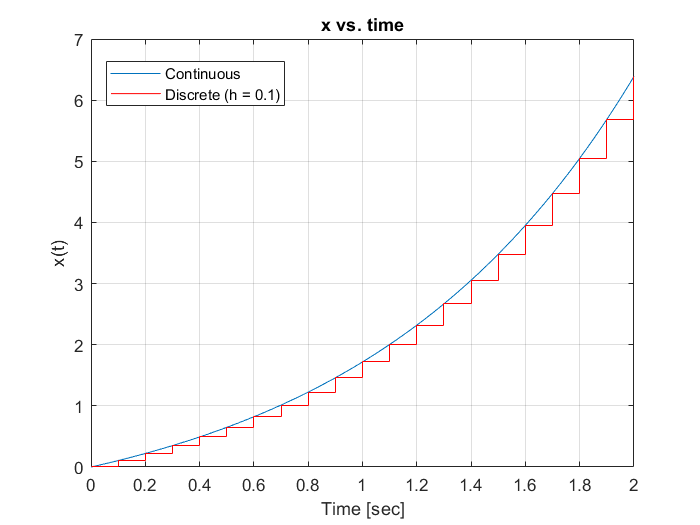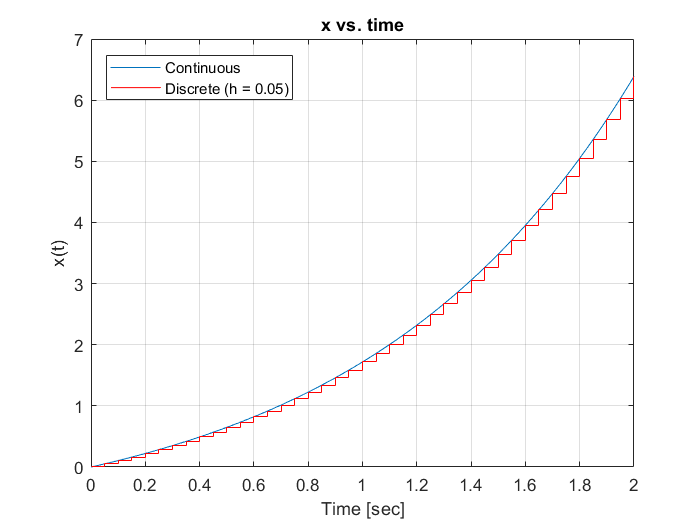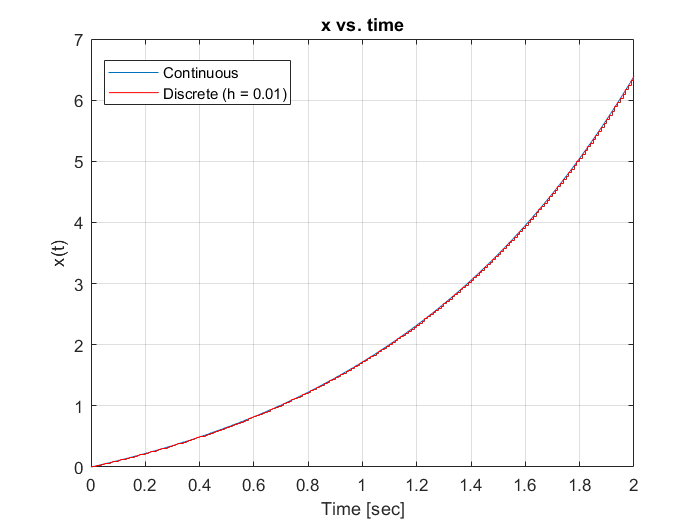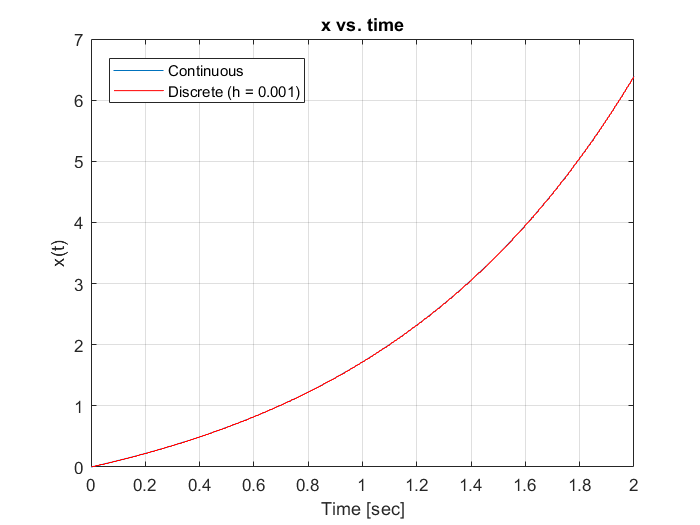So one can see that $h$ reduces, the discrete-time response comes closer to that of the continuous-time response.

The results derived for a specific dynamic system in this note can be generalized for any linear dynamic system in any number of dimensions. An interested reader may attempt to do so and post his/her comments on this subject.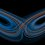Note by Karan Chatrath
1 year, 11 months ago

This discussion board is a place to discuss our Daily Challenges and the math and science related to those challenges. Explanations are more than just a solution — they should explain the steps and thinking strategies that you used to obtain the solution. Comments should further the discussion of math and science.

When posting on Brilliant:

• Use the emojis to react to an explanation, whether you're congratulating a job well done , or just really confused .
• Ask specific questions about the challenge or the steps in somebody's explanation. Well-posed questions can add a lot to the discussion, but posting "I don't understand!" doesn't help anyone.
• Try to contribute something new to the discussion, whether it is an extension, generalization or other idea related to the challenge.
• Stay on topic — we're all here to learn more about math and science, not to hear about your favorite get-rich-quick scheme or current world events.

MarkdownAppears as
*italics* or _italics_ italics
**bold** or __bold__ bold
- bulleted- list
• bulleted
• list
1. numbered2. list
1. numbered
2. list
Note: you must add a full line of space before and after lists for them to show up correctly
paragraph 1paragraph 2

paragraph 1

paragraph 2

[example link](https://brilliant.org)example link
> This is a quote
This is a quote
    # I indented these lines
# 4 spaces, and now they show
# up as a code block.

print "hello world"
# I indented these lines
# 4 spaces, and now they show
# up as a code block.

print "hello world"
MathAppears as
Remember to wrap math in $$ ... $$ or $ ... $ to ensure proper formatting.
2 \times 3 $2 \times 3$
2^{34} $2^{34}$
a_{i-1} $a_{i-1}$
\frac{2}{3} $\frac{2}{3}$
\sqrt{2} $\sqrt{2}$
\sum_{i=1}^3 $\sum_{i=1}^3$
\sin \theta $\sin \theta$
\boxed{123} $\boxed{123}$

## Comments

Sort by:

Top Newest

It's interesting that you introduced exponentials into this. My basic intuition would have been:

$\dot{x} = x + u \\ x(T+h) = x(T) + h \dot{x} (T) \\ x(T+h) = x(T) + h \Big( x(T) + u(T) \Big) \\ x(T+h) = x(T) (1 + h) + h u(T)$

Of course, as we know from numerical integration in general, there are a variety of ways to do the computations. With a sufficiently small step-size, they should all basically agree.

- 1 year, 10 months ago

Log in to reply

Hello! It is true that approximating the derivative is a more straightforward approach to discretization. You rightly pointed out that there exist many approaches to go about this operation and that with a sufficiently small step size, the response would be indistinguishable with the continuous-time response.

A good way to compare these methods is by doing so in the frequency domain. Certain methods lead to a discrete system which approximates the frequency response better than other discretization methods.

The method described in this note is in fact, not the best approach when one considers frequency domain responses. It is an interesting approach though.

- 1 year, 10 months ago

Log in to reply

I was thinking about that. This reminds me of the 2-tap vs 3-tap differentiator exercise. Would you like to post a problem comparing the frequency response of your method vs. the Euler-style approach?

- 1 year, 10 months ago

Log in to reply

Thanks for the suggestion. I will think of a problem and post it.

- 1 year, 10 months ago

Log in to reply

I have posted a problem in the calculus section. I feel that it is worded in a slightly convoluted manner but I've tried my best to be clear. Please give suggestions if necessary.

- 1 year, 10 months ago

Log in to reply

That was a nice problem. Thanks for posting it. I will post a solution a bit later today when I have some more time.

- 1 year, 10 months ago

Log in to reply

×

Problem Loading...

Note Loading...

Set Loading...